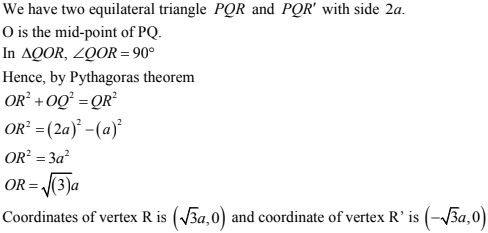# Chapter 14 Co-Ordinate Geometry RD Sharma Solutions Exercise 14.1 Class 10 Maths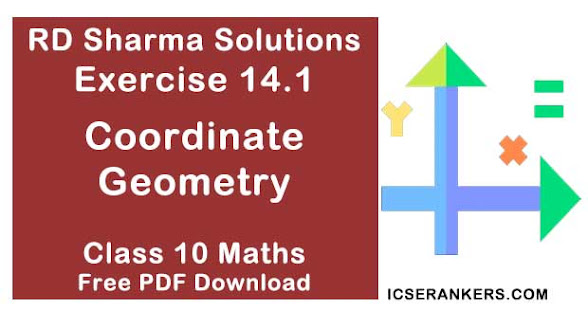Chapter Name RD Sharma Chapter 14 Co-Ordinate Geometry Book Name RD Sharma Mathematics for Class 10 Other Exercises Exercise 14.2Exercise 14.3Exercise 14.4Exercise 14.5 Related Study NCERT Solutions for Class 10 Maths

### Exercise 14.1 Solutions

1. On which axis do the following points lie?
(i) P(5, 0)
(ii) Q(0 – 2)
(iii) R(−4, 0)
(iv) S(0, 5)

Solution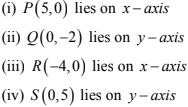2. Let ABCD be a square of side 2a. Find the coordinates of the vertices of this square when
(i) A coincides with the origin and AB and AB and coordinate axes are parallel to the sides AB and AD respectively.
(ii) The center of the square is at the origin and coordinate axes are parallel to the sides AB and AD respectively.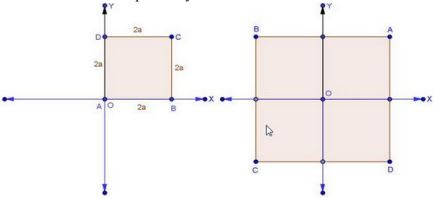Solution

(i) Coordinate of the vertices of the square of side 2a are :
A(0, 0), B(2a, 0), C(2a, 2a) and D(0, 2a)
(ii) Coordinate of the vertices of the square of side 2a are :
A(a, a), B(-a, a), C(-a, -a) and (a, -a)

3. The base PQ of two equilateral triangles PQR and PQR’ with side 2a lies along y-axis such that the mid-point of PQ is at the origin. Find the coordinates of the vertices R and R’ of the triangles.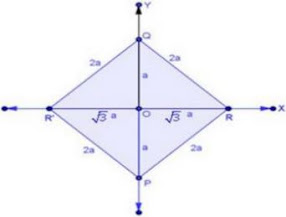Solution